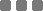## Wednesday, November 23, 2016

### Math: How to Convert Decimal Point Thing to Fraction

Non-repeating Decimal

0.05 to Fraction

0.05 can be written as \$5/100\$

\$5/100\$ can be reduced by finding the GCF (Greatest Common Factor) from 5 and 100, which is 5. Then divide both numerator (the top fraction part: 5) and denominator (bottom part: 100) with 5.

\$0.05 = 5/100 = 5/100 : (5/5)\$
\$(5 : 5)/(100 : 5) = 1/20\$

So, \$0.05 = 1/20\$

0.65 to Fraction

0.65 can be written as \$65/100\$

\$65/100\$ can be further simplified by finding the GCF, 5. And then we divide both fraction parts with 5.

\$0.65 = 65/100 = 65/100 : (5/5)\$
\$(65 : 5)/(100 : 5) = 13/20\$

Therefore, 0.65 is \$13/20\$ in fraction format.

3.175 to Fraction

3.175 can be written as \$3 + 0.175\$, convert the decimal point part to fraction ► \$3 + 175/1000\$

So we have a mixed fraction \$3 175/1000\$.

\$3.175 = 3 + 0.175\$
\$3 + 175/1000 = 3 + (175/1000 : (25/25))\$
\$3 + (175:25)/(1000:25) = 3 7/40\$

\$3.175 = 3 7/40\$

Repeating Decimal

0.111111... to Fraction

We have one-pattern repeating sequence (1).

This uses a bit of algebra to convert it to fraction.

Let's take \$n = 0.111111...\$

"Shift" the n value one digit to right: \$10n = 1.111111...\$

Now, if we subtract 10n and n, the result will be an integer (both for n coefficient and the value).

\$10n - n = 1.1111... - 0.1111...\$

\$9n = 1\$

\$n = 1/9\$

There you go, \$0.11111111.... = 1/9\$

1.23123123123... to Fraction

We have three-pattern repeating sequence (123).

Before we do the earlier method, first, observe the number before shifting some digits to right or left. These examples only emphasize the "right shift".

Let's take \$n = 1.23123123123...\$

We shift the point part 3 digits more to the right (multiply with 1000): \$1000n = 1231.23123123...\$
Again, same like above, if we subtract 1000n and n, it will produce an integer (both sides).

\$1000n - n = 1231.23123123... - 1.23123123...\$

\$999n = 1230\$

\$n = 1230/999\$

Apparently 1230 and 999 have 3 as the GCF. Divide both with it to simplify the fraction.

\$n = 1230/999 : (3/3) = (1230:3)/(999:3) = 410/333\$

Or, we can write the improper fraction: \$410/333\$ as mixed fraction ► \$1 77/333\$

So, \$1.23123123123... = 410/333 = 1 77/333\$

15.65415654156541... to Fraction

We have five-pattern repeating sequence (15654).

Let \$n = 15.6541565415654...\$

Shift it 5 digits to right (multiply with \$10^5\$), so: \$100000n = 1565415.65415654...\$

\$100000n - n = 1565415.65415654... - 15.65415654...\$

\$99999n = 1565400\$

\$n = 1565400/99999\$

\$15.65415654156541... = 1565400/99999 = 15 65415/99999 = 15 (65415:3)/(99999:3) = 15 21805/33333\$ (no further reduction)

Now, for repeating decimal, it can be in mixed forms, like, the whole number isn't "repeating", but the number behind the point has repeating pattern. For instance: 10000.543215432154321..... or 509.9999999... or 29.787878787... or maybe like 0.000234500123123123123... and such others.

Yea, that's about it. A glimpse. I'm sure you can expand the basic idea to do your own stuff.

The important things to remember when converting repeating decimal to fraction:
1. Spot the repeating pattern. You can use underline or highlighter or something to mark each pattern.
2. Then do the algebra accordingly.

All in all, this is about numero. The language of the angels[citation needed].

If you forgot how to find GCF or LCM, go to this linkMath: How to Convert Decimal Point Thing to Fraction
https://monkeyraptor.johanpaul.net/2016/11/math-how-to-convert-decimal-point-thing.html